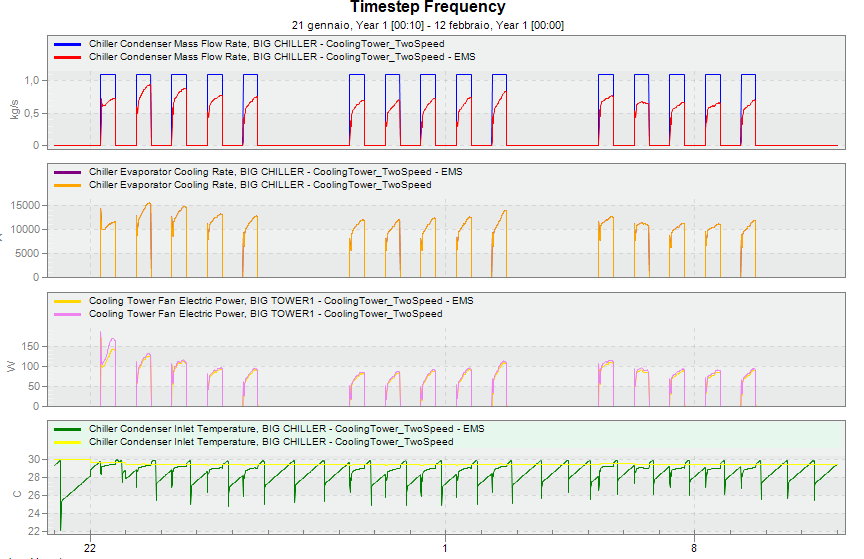Question-and-Answer Resource for the Building Energy Modeling Community
Get started with the Help page

Condenser water flow rate with EMS: EnergyPlus

My question is related to this issue (https://unmethours.com/question/35540... ticket: https://github.com/NREL/EnergyPlus/is...) where the condenser pump is not working as expected.

Is there a way to control the condenser water flow rate with an EMS program? I guess it could be done using the "Chiller Condenser Heat Transfer Rate [W]" output as sensor and a "SetpointManager:Scheduled" as actuator. Unfortunately it is not possible since the condenser loop requires a temperature setpoint.

Is there another way?

edit retag close merge delete

Sort by » oldest newest most voted

There is an actuator for pumps called Pump Mass Flow Rate [kg/s] (cf EMS Application Guide) that you should use for this.

Chiller Condenser Heat Transfer Rate [W] as a sensor would probably work if you have only one chiller on the demand side of your condenser loop. Otherwise you can probably use the Plant Supply Side Cooling Demand Rate [W] as a sensor.

You'll probably also need to get the Entering Water Temperature (EWT) too, for eg looking at Plant Supply Side Inlet Temperature. If your SetpointManager:Scheduled isn't using a fixed value that you can hardcode in your EMS program, you also need to define a Sensor on the Schedule Value for the corresponding schedule, that will give you the Leaving Water Temperature (LWT).

I think Plant Supply Side Cooling Demand Rate is a positive number, in which case I guess you could try this:

$$\dot{Q}_{demand} = \dot{m} \cdot C_p \cdot (T_{EWT} - T_{LWT})$$

Dimensional analysis:

$$[W = J \cdot s^{-1}] = [kg.s^{-1}] \cdot [J.kg^{-1}.K^{-1}] \cdot [K]$$

So, solving for your mass flow rate:

$$\Leftrightarrow \dot{m} = \frac{\dot{Q}_{demand}}{C_p \cdot (T_{EWT} - T_{LWT})}$$

You can use @CpCW(Temperature) in ERL if you'd like, but it's pointless since it'll always return 4180.0 anyways (source code: Psychrometrics.hh)

Disclaimer: I have not tested any of the above, not sure whether it'll work, integrate well with what the cooling tower is doing, nor what calling point would be appropriate.

more

uh yes, those equations are exactly what I was thinking about: the pump actuator is what I was missing. I guess I will try with a fixed leaving water temperature at 30°C and using the entering water temperature as sensor. I'm going to try it as soon as possible and I'll post some results here.

I think it works....! Thanks a lot, I'll add the results in another answer below.

Following the previous answer by Julien Marrec I tried the following:

1. I used the example file "CoolingTower_TwoSpeed"
2. Run period 01/05 to 20/05 and Weather file "USA_CA_San.Francisco.Intl.AP.724940_TMY3.epw"
3. I created a model with EMS called "CoolingTower_TwoSpeed_EMS"
4. I added a sensor using the variable "Plant Supply Side Cooling Demand Rate" with the name "PSSCDR"
5. I added an actuator on the pump variable "Pump Mass Flow Rate" with the name "PMFR"
6. I added a simple program with the line "set PMFR=PSSCDR/4180/5+1.1*0.025"

Note that it is a very rough control: I used a fixed temperature difference of 5 degrees. The main problem is that with this method the plant temperatures are getting too hot during the warm-up and you will get an error. Therefore I added a constant value equal to the 2.5% of the design pump mass flow rate (1.1 kg/s): it solved the temperatures error in my case.

I obtained: -21.88% pump energy consumption and -1% in cooling energy consumption (beacause the chiller condensed inlet temperature is lower in the EMS case). The energy saving is related to this specific example.more